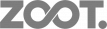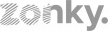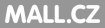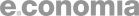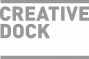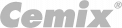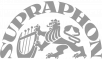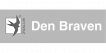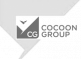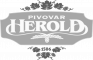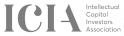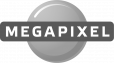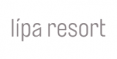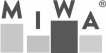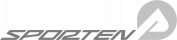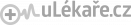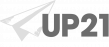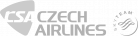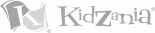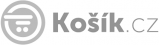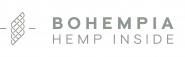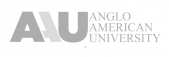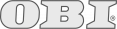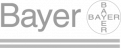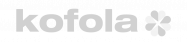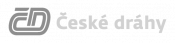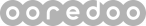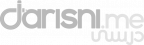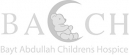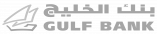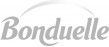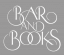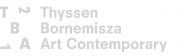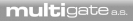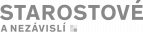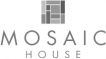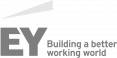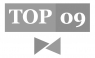s t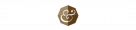E X P E R I E N C E   T H E M . "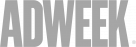C A N   I N C R E A S E   Y O U R   R E S P O N S E   U P   T O   3 0 0 %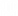J O I N   T H E S E   G R E A T   B R A N D S   W E   W O R K E D   F O R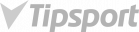T H E R E ' S   A   P L A C EF O R   Y O U R   B R A N D